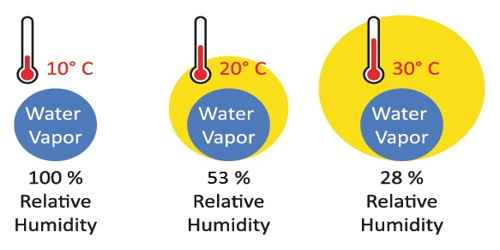Physics

# Relative HumidityRelative humidity: It is defined as the ratio of the mass of water vapour present in a given volume of air at a particular temperature to the mass of water vapour required to saturate the same volume of air at the same temperature. It expressed commonly as a percentage value, is the ratio of the actual amount of water vapour present in a sample (the Absolute Humidity) to that amount that would be needed to saturate that particular sample.It expresses the degree of wetness of the atmosphere air. It is denoted by R.

Relative humidity, R = (Mass of water vapour in a given volume of air/Mass of water vapour)

Since the mass of water vapour is proportional to its pressure, we can write,

R = (Pressure of water vapour in air / Saturated vapour pressure at air temperature)

Further, we know that the water vapour present in air is equal to the saturated vapour pressure at dew point.

R = (Saturated vapour pressure of water at dew point / Saturated vapour pressure of water at air temperature)

Relative humidity, R is usually expressed in percentage,

R = f/F x 100%

Here f is the saturated vapour pressure of water at dew point and F, the saturated vapour pressure of water at air temperature.

The relative humidity of an air-water mixture is defined as the ratio of the partial pressure of water vapour (H2O) in the mixture to the saturated vapour pressure of water at a given temperature. Thus the relative humidity of air is a function of both water content and temperature.